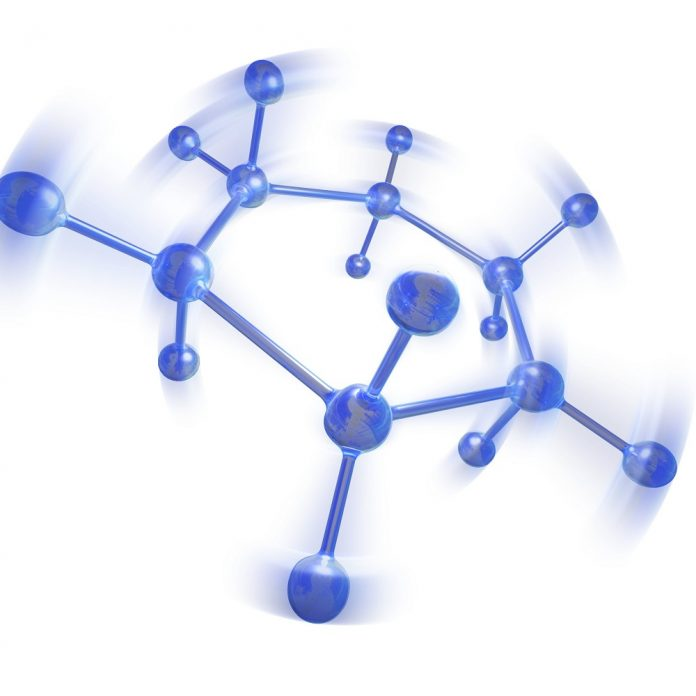The chemistry which deals with different states of matter is solid-state chemistry. It is also called as material chemistry which demonstrates the structure and properties of solids. Matter can exists in three states- solid, liquid and gas. Here solid state deals with the state solid, their types and properties.

Solid-state is one of the three basic states of matter which is formed from liquid or gas. The molecules that make up a solid are arranged in regular and repeating patterns. It exhibits certain Characteristics that distinguish them from liquid and gases.

## Physical and Chemical properties of Solids

• Solids have definite shape and volume.
• They are rigid and unable to compress easily
• They are having high density and heavy
• They don’t have the ability to flow and intermolecular force of attraction will be more
• The particles can oscillate in their mean positions.

## Types of Solids

There are two types of solids

1. Crystalline solids
2. Amorphous solids

## Crystalline Solids

Crystalline solids have a high degree of order in a periodic arrangement. The majority of solids are crystalline solids as they are having a definite geometrical arrangement of atoms. The crystalline solids are considered as true solids.

### Properties of Crystalline Solids.

• These type pf solids have a particular three-dimensional geometrical structure.
• The atoms or ions in these type of solids are arranged in long order.
• The melting point of crystalline solids is sharp.
• The crystalline solids have uniform chemical composition throughout.
• They have specific characteristic orientation and bounded by a flat surface.
• Example: Common table salt belongs to crystalline solids

## Amorphous solids

Amorphous solids are non-crystalline solids in which the atoms and molecules are not arranged in a definite lattice pattern. It lacks an ordered internal structure.

### Properties of Amorphous solids

• The strength of the different bond is different in this type of solids.
• The intermolecular force is weaker than that of crystalline solids.
• They do not have a proper structure and do not have sharp melting points.
• Amorphous solids are said to be isotropic.
• Examples: Rubber, plastics and gels.

# Van Der Waal Equation

In 1873 Johannes Diderik Van Der Waals, a Dutch physicist postulated Van Der Waal forces to account real gases. Van Der Waals force is an attraction of intermolecular force between molecules. It is a weak electric force that attracts neutral molecule and much weaker than chemical bonds.

The van der Waals equation is an equation stated for real gas and is given by,

(P+an2/V2)(V-nb)

Where,

P = Pressure

V = Volume

T = Temperature

n for the number of moles

. ‘a’ and ‘b’ are constants.

## Ideal Gas Equation and Van Der Waals Equation

The ideal gas equation is

Pv = nRT

Where,

P is pressure

V is volume

T is temperature

n is the number of moles and  R is the universal gas constant

Van Der Waals Equation is

(P+an2/V2)(V-nb)

Van Der Waal Equation for real gases is the corrected form of the ideal gas equation and is given by

(P+an2/V2)(V-nb) = nRT

Where a and b are constants

The constant ‘a’ accounts for the fact that there is the intermolecular force between molecules and the constant ‘b’ accounts for the fact that molecules occupy some space of the container.

an2/V2  and nb correct the pressure and volume in the ideal gas equation respectively.

## Summary

• Solid-state is a state of matter which deals with the study of solids, their types and properties.
• There are two main types of solids: crystalline and amorphous. In crystalline solids, atoms are arranged in long order and amorphous solids are disordered.
• The van der Waals equation is an equation of state that corrects for two properties of real gases: the excluded volume of gas particles and attractive forces between gas molecules.
• Van Der Waals Equation for real gas is given by, (P+an2/V2)(V-nb)